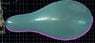# How to determine volume from a picture?

skrat
Hello,

It's my first time here on this forum and if my English is bad, than I at least hope I have chosen the right topic.

Well, let me describe my problem: Imagine a .jpg with a balloon. What I have to do is determine a volume of it in the moment before it blows up. So in reality I have a movie, but I used a program where I can move frame by frame to get the last frame before the balloon explodes.

Now, how can I determine the volume from surface? I tried to use this http://getdata-graph-digitizer.com/ , a program that can export .txt file of coordinates. But when I put this .txt file into "homemade" program written in Java to calculate the proper integral, the volumes don't make any sense and I am 100% sure that my program works properly and I even checked the units 25 times.

Is there any other way to do is more elegant and as precise as possible? (Let's say that balloon is totally symmetrical).

#### Attachments

•Capture.JPG
22.4 KB · Views: 386

Homework Helper
When you say "surface" you mean the surface seen projected onto the plane, not the surface of the three dimensional balloon, right? Let's start with a simpler version. A sphere, with radius R, has volume $(4/3)\pi R^3$ and projects onto any plane as a circle of radius R, having area $\pi R^2$. Therefore the volume is $((4/3)\pi R^3)/(\pi R^2)= (4/3)R$ times the area.

For the more complicated version, approximate the volume as a sum of spheres of differing radii.

skrat
When you say "surface" you mean the surface seen projected onto the plane, not the surface of the three dimensional balloon, right?

Yes, I mean surface seen projected onto the plane.

hmmm... It's a good idea. But the problem is that I have to calculate the volume of 20 balloons. As you probably know, the difference between the volumes before explosion should be zero if the balloons were ideal, since they are not, I expect some difference. However, I am afraid that this approximation with spheres is not good enough to "detect" the small difference, unless of course , I try to minimize the spheric radius as much as possible.

Your idea works in theory, but honestly said, I have no idea how to write that in java or c+.

Edgardo
Do you mean by surface the purple curve? If yes, you could rotate this curve around the "x-axis" as shown here. You would have to adjust the jpeg to make the balloon symmetrical around the x-axis.

The idea is to http://calculus-geometry.hubpages.com/hub/Disk-Method-Volume-of-Solid-of-Revolution [Broken] to get the volume.

Last edited by a moderator:
Number Nine
Using the grid, you could select some representative points on the boundary of the balloon (say, on the pink like) and fit a curve to them. Rotating the curve around the x-axis by the usual integration should give you a pretty good approximation.

skrat
Do you mean by surface the purple curve? If yes, you could rotate this curve around the "x-axis" as shown here. You would have to adjust the jpeg to make the balloon symmetrical around the x-axis.

The idea is to http://calculus-geometry.hubpages.com/hub/Disk-Method-Volume-of-Solid-of-Revolution [Broken] to get the volume.

Well yes, that is exactly what I did. This purple curve is full of points which I can manually put there with the program written above (Graph Digitzer). This program than exports X and Y coordinates of all the points in .txt file.
Later I used my own program written in .java to calculate the integral: $$V=\pi \int_{a}^{b}f(x)^{2}dx$$ where for $$f(x)=\frac{f(x_{n+1})+f(x_{n})}{2}$$ and $$dx=x_{n+1}-x_{n}$$

I hope this sounds good?

I was just wondering if there is a better method to do this? Because for this, I had to use a lot of photoshop to rotate the picture until the balloon was perfectly horizontal ( because all balloons were not straight - see attachment) in order to get the integral as precise as possible. If anybody is thinking that was silly, well he is right, I could simply define new coordinate system but, sadly software I used - Graph Digitizer, does not have that option and even if it did, it would be extremely hard to determine units.

So is there a simpler and faster version how to do this with the same or even better precision. Fitting a curve? Well yes, another option too, but the fact is that the difference between the volumes is so small that the software might use the same functions for every balloon - meaning the same volume for all of them

#### Attachments

Last edited by a moderator: